# Primal Digital Medium

AUTHOR: Samuel Pietri
UPDATED:

The paper How a life-like system emerges from a simple particle motion law by Thomas Schmickl, Martin Stefanec & Karl Crailsheim describes a simple motion law for moving and interacting particles leading to a self-structuring, self-reproducing and self-sustaining life-like system. That’s the way they describe it in the article and it definetily cought my attention. These emerging patterns look like really basic organism. Some structures emerge and grow over time while the particles interact with each other. So I was interested in implementing the system in a simulation program and explore its aesthetic potential. For all the technical detail make sure you read the article. I’ll just outline the main framework of the setup leaving most of the detail for further readings. So unlike other simple computational models, such as cellular automata, this system is able to produce natural-like structures and dynamics without any restriction to unnatural modeling constraints. The particles respect only this motion rule, described by this pseudo code here:

``````loop foreach timestep{
loop foreach particle{
L = (count other particles in left semicircle with radius r)
R = (count other particles in right semicircle with radius r)
N = L + R
delta_phi = alpha + beta * N * sign(R - L)
rotate delta_phi # positive values: rotate to the right
move-forward v
}
}

``````

Each particle determines the number of its neighbors on the left side (all the particles inside a semicircle with radius r) and on the right side(all the particle inside the other semicircle of radius r). The change of its heading angle (delta_phi) every time step is the responsible of the particle’s motion. After the rotation each particle moves forward with a fixed velocity.

The key formula to make the system working properly is the one that calculates the angle of rotation.They discovered an interesting system with the parameter set:

``````r =5
alpha = 180°
beta = 17°
v = 0.67

with a density(particles/space unit) of 0.08
``````

In this situation structures similar to cell start to form randomly from the particle soup.

Given these information I then implemented the algorithm in openFrameworks. Starting from the particle system I used previously for strange attractors post I then added the magical rule inside the fragment shader so where I update the particle’s position and velocity.

Here’s the fragment code for the force.

``````vec4 primalForce(vec3 p, vec3 v){

ivec2 texSize = textureSize(particles0);
float angle = 99999999999999.0f;

if(v.x != 0) angle = atan(v.y/v.x);

vec3 primeForce = vec3(0.0, 0.0, 0.0);

float speed = inSpeed;

int R = 0;
int L = 0;
int N = 0;

// Here I assign the value of the distance threshold for the neighbor search

for (int x = 0; x < texSize.x; x ++){
for (int y = 0; y < texSize.y; y++) {

float otherAngle = 99999999999999.0f;

vec3 pos = texture(particles0, vec2(x, y)).xyz;
vec3 otherVel = texture(particles1, vec2(x, y)).xyz;

float d = distance(p, pos);

if (d > 0 && d< primeRadius){

vec2 offsetPosition = vec2(pos-p);
float xx = offsetPosition.x * cos(-angle) - offsetPosition.y * sin(-angle);
float yy = offsetPosition.x * sin(-angle) + offsetPosition.y * cos(-angle);
//
if(xx != 0)  otherAngle = atan(yy/xx);
//
if (otherAngle >= 0) {
L++;
}
else {
R++;
}

}
N = int(L+R);
}
}

// Key formula
float delta_phi = alpha + beta * N * sign(R - L);

angle += delta_phi;

float newAngle = angle;

if(newAngle <= -PI) newAngle += 2*PI;
if(newAngle > PI) newAngle -= 2*PI;
angle = newAngle;

// value for display purposes
float nMapped = map(N, 0, 100, 0.f, 1.0f);
vec4 velocity = vec4(cos(angle) * speed, sin(angle) * speed, 0.0, nMapped);

return velocity;

}
``````

This force, then added to the velocity value, is the main responsible for the particle motion.

I then tested the simulation changing the key parameters discussed above and here below you can see a few images extracted from these tests.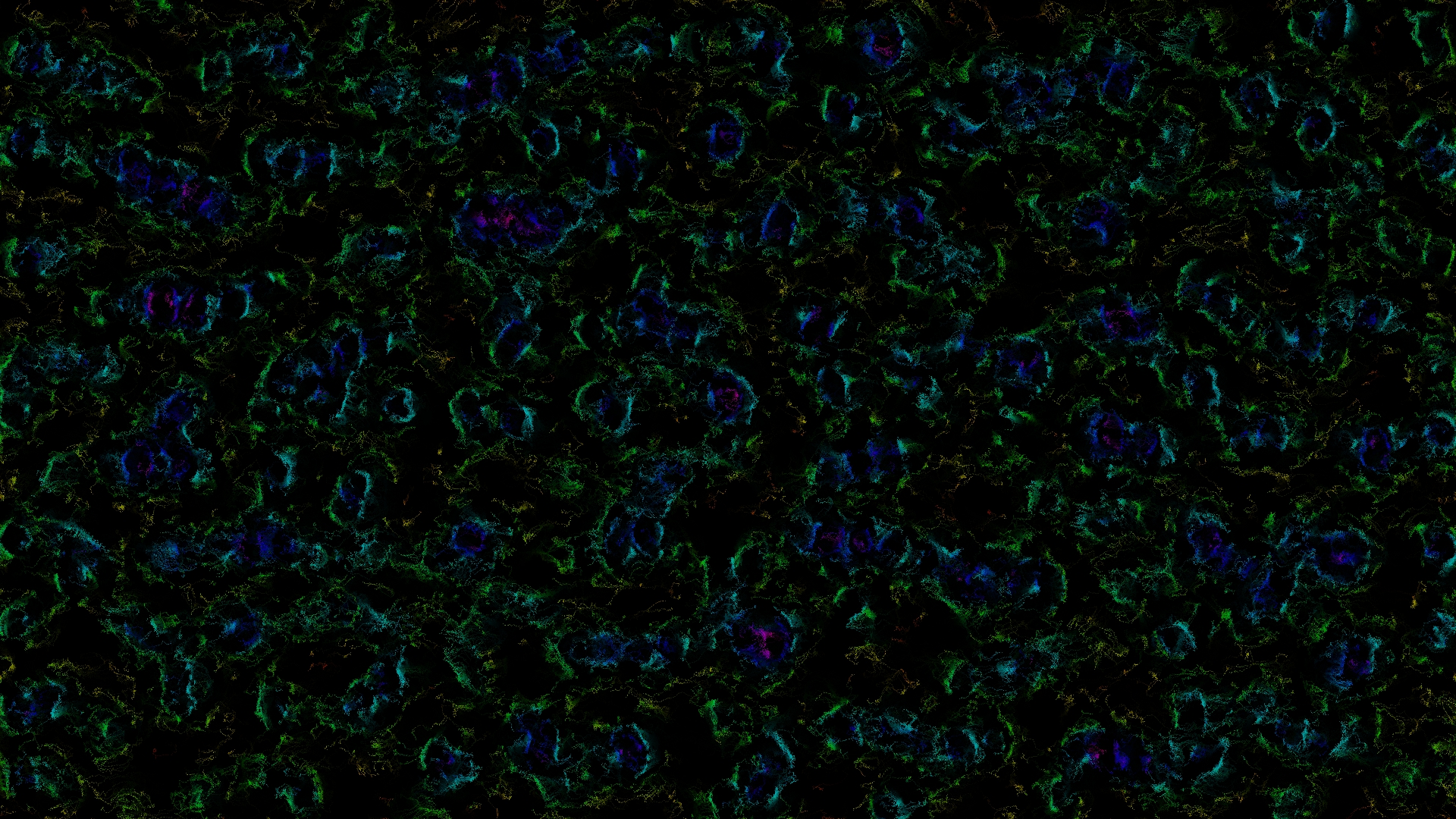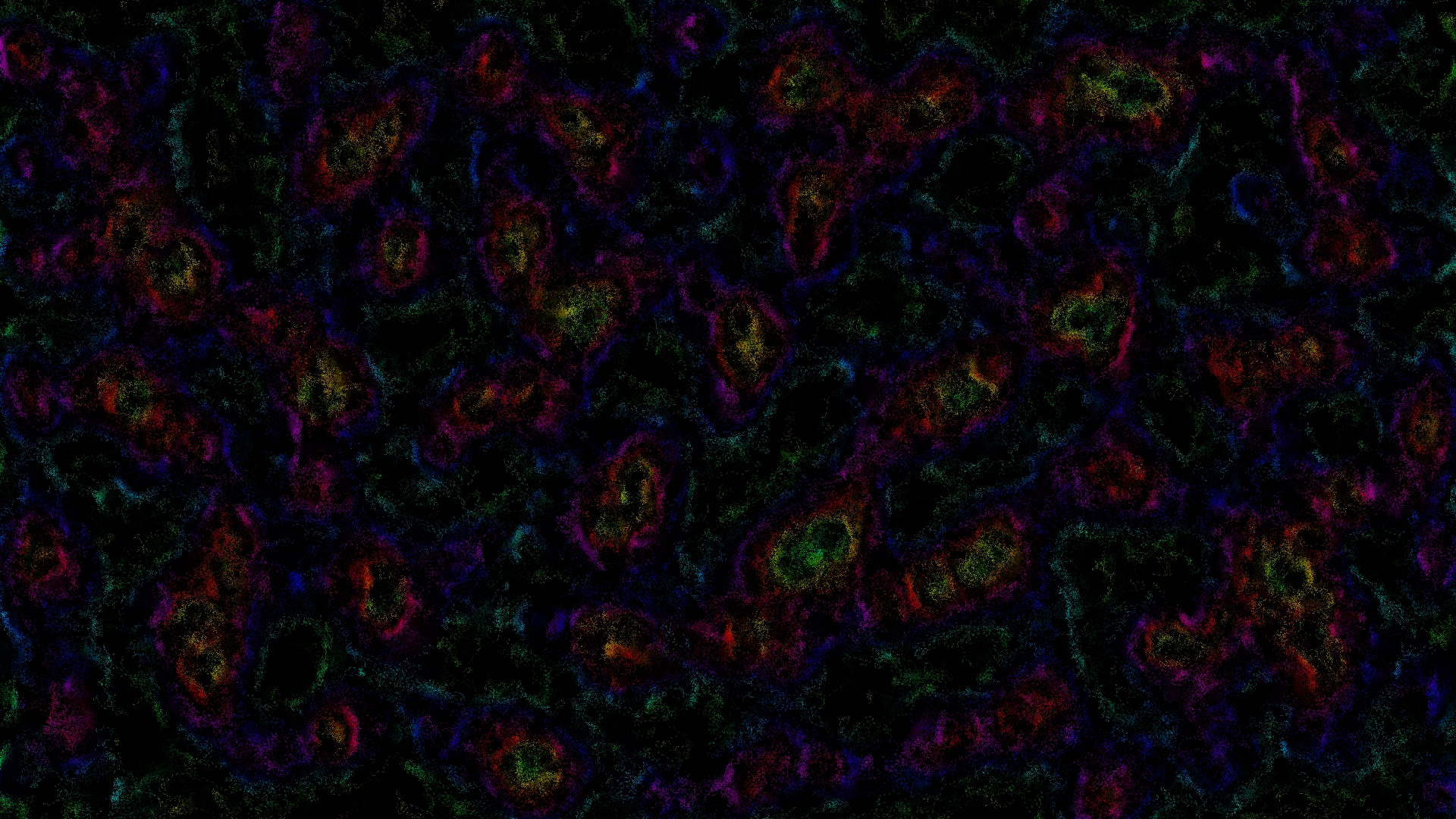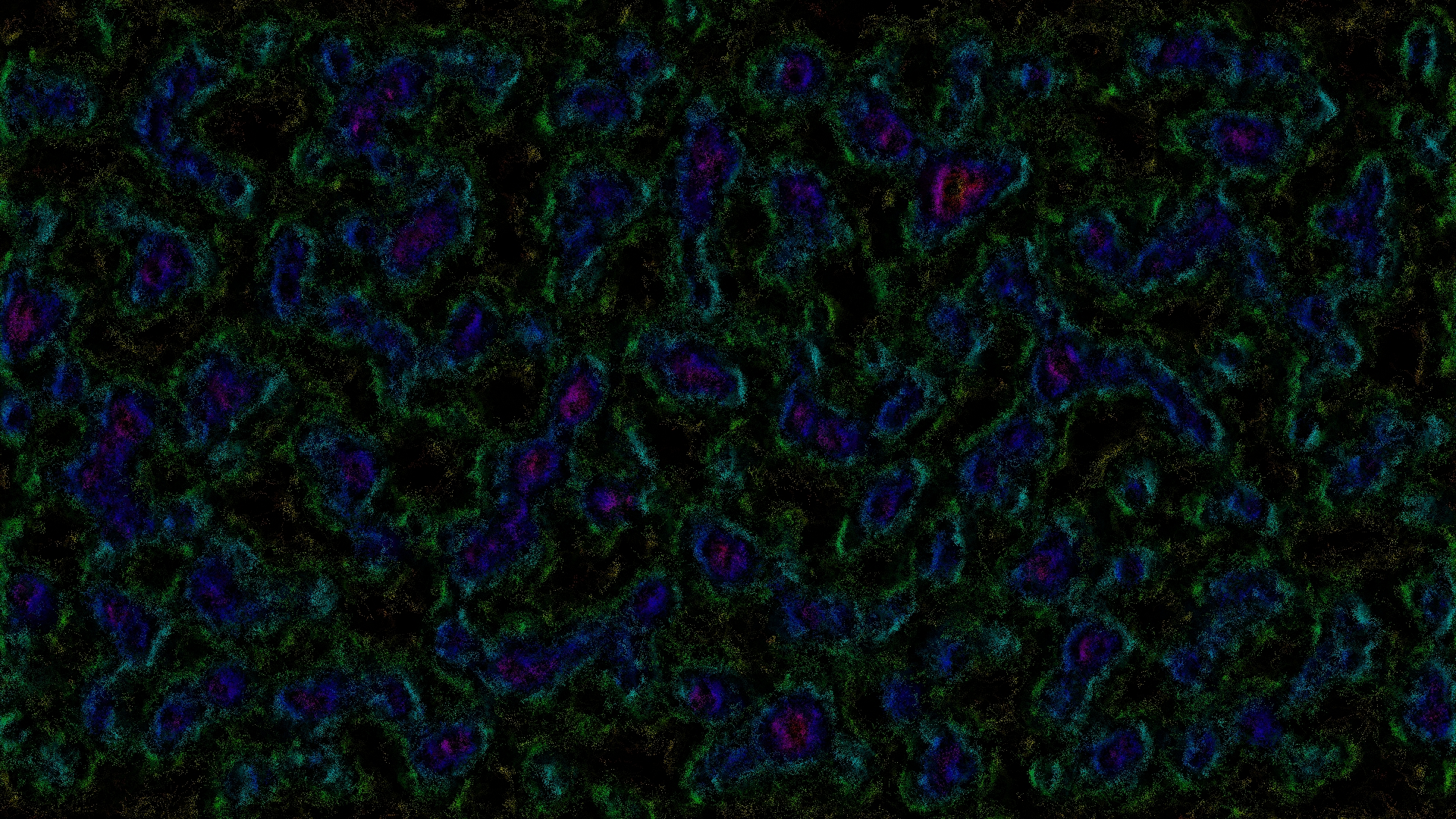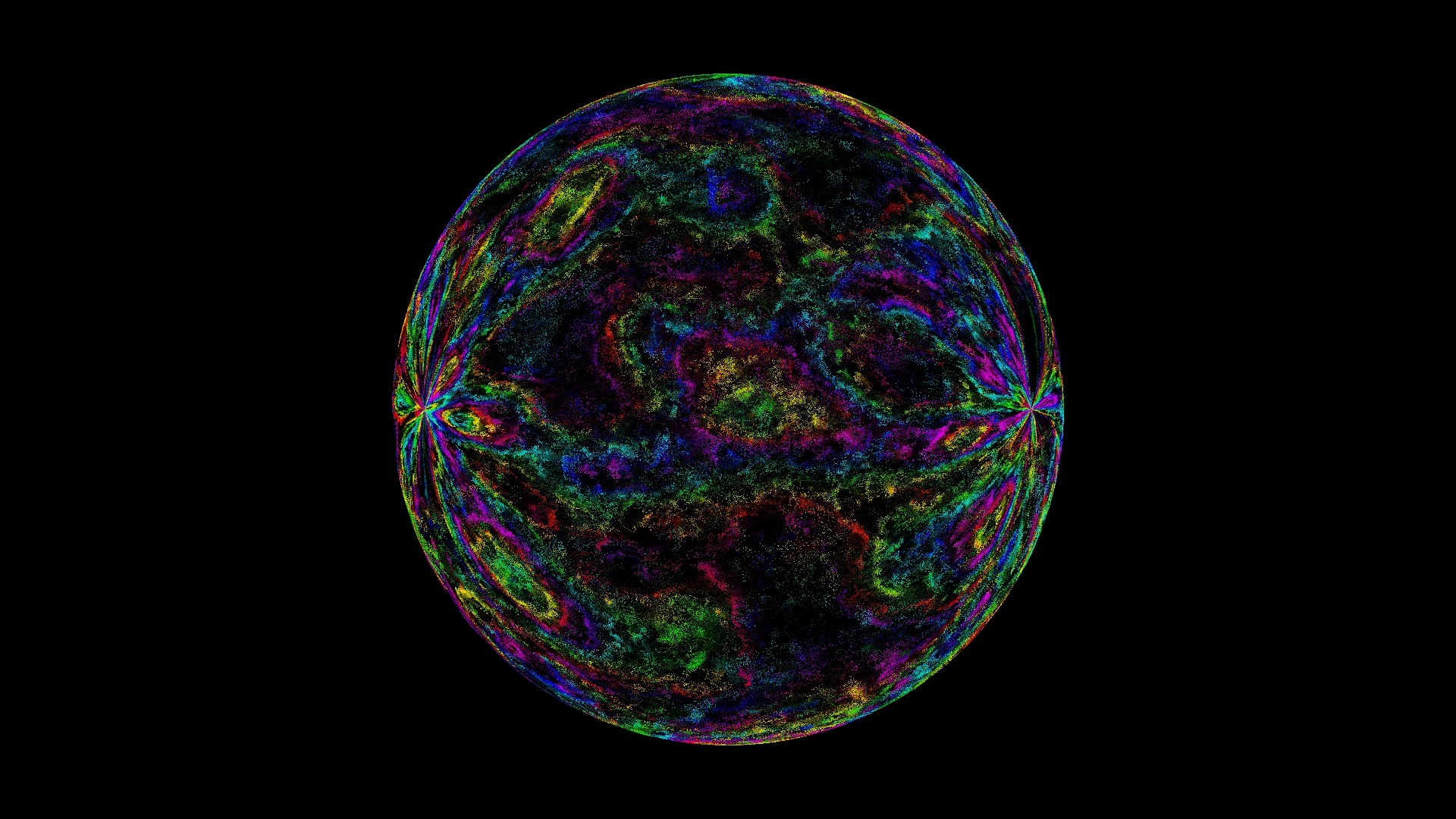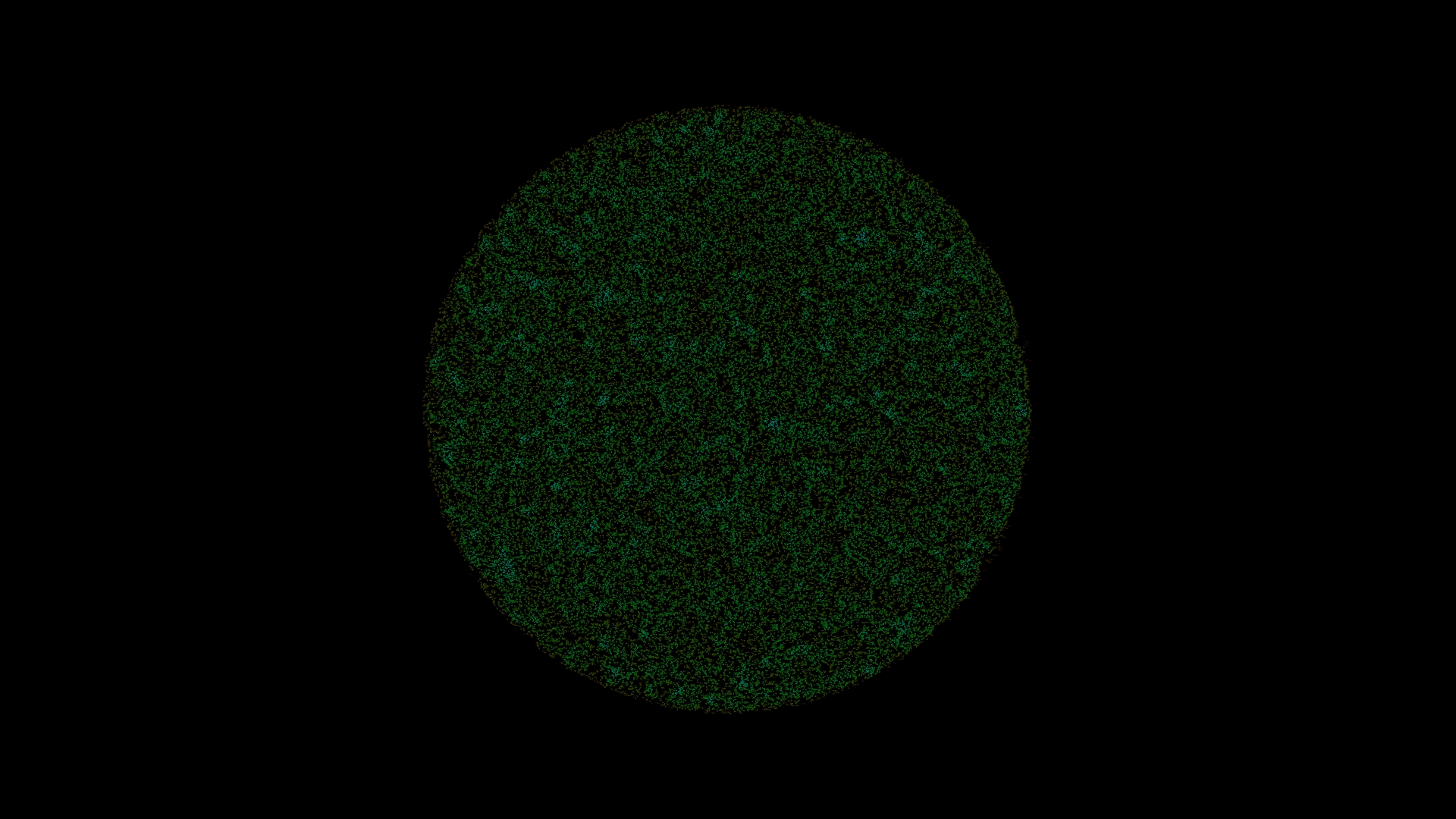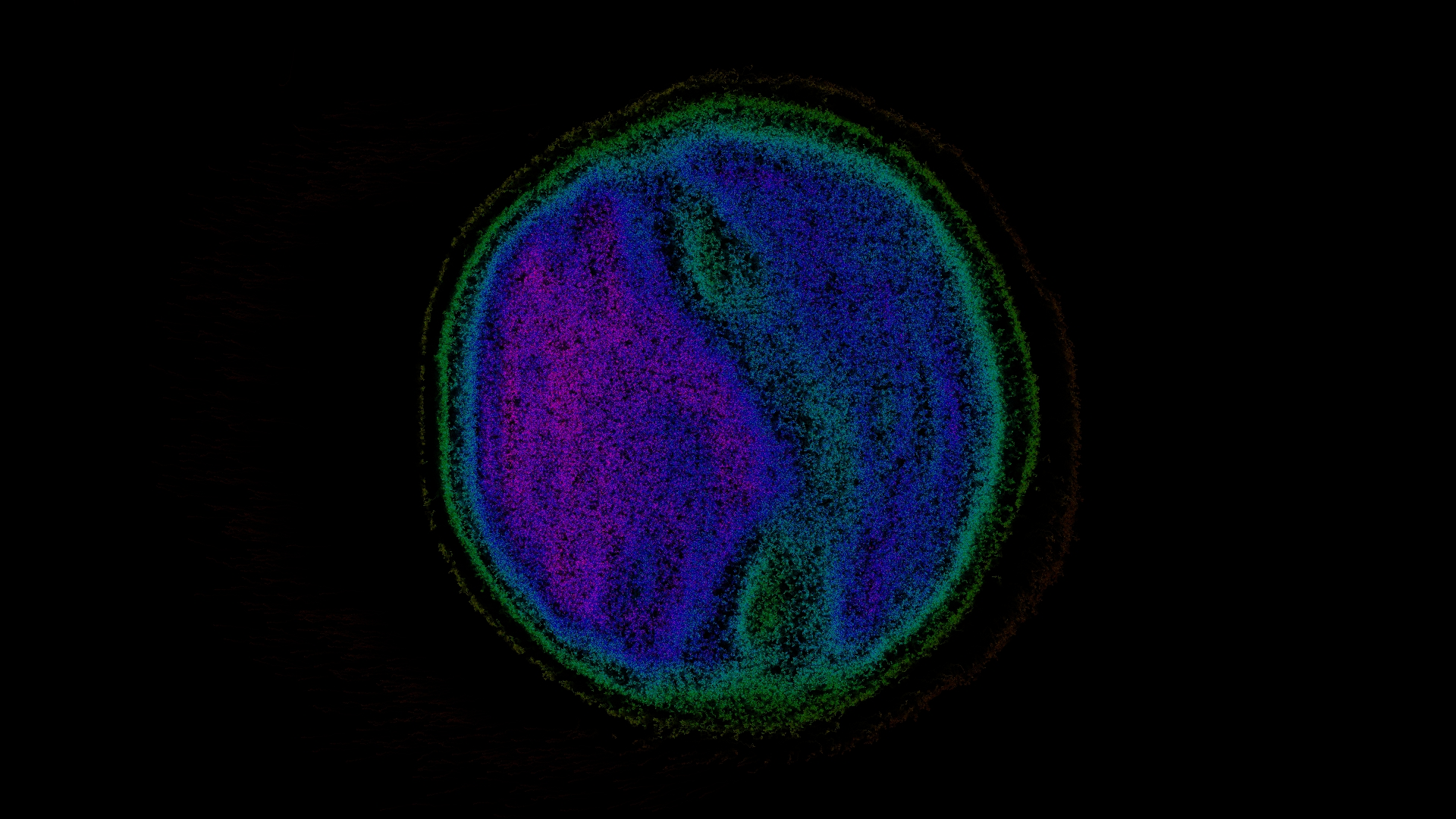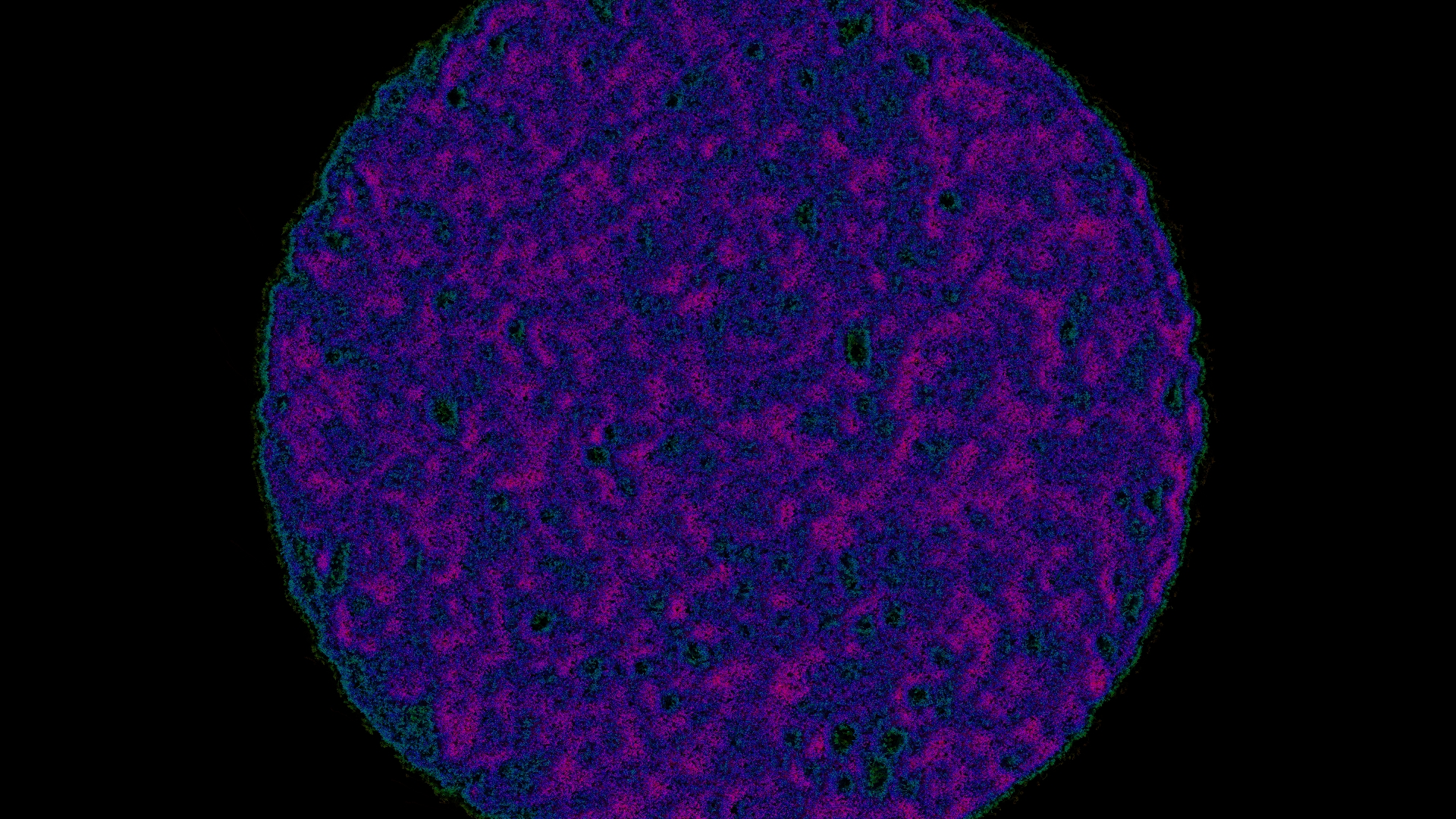Tags:

Categories: